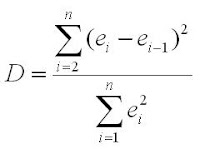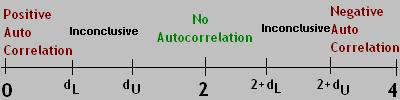## Tuesday, March 4, 2008

### Lecture 8 - Residual Analysis - Checking Independence of Errors

Checking the Independence of Errors Assumption
The "I" in the LINE mnemonic stands for Independence of Errors. This means that the distribution of errors is random and not influenced by or correlated to the errors in prior observations. The opposite is independence is called autocorrelation.

Clearly, we can only check for independence/autocorrelation when we know the order in which the observations were made and the data points were collected.We check for independence/autocorrelation in two ways. First, we can plot the residuals vs. the sequential number of the data point. If we notice a pattern, we say that there is an autocorrelation effect among the residuals and the independence assumption is not valid. The plot at right of residuals vs. observation week shows a clear up and down pattern of the residuals and indicates that the residuals are not independent.

The second test of independent/autocorrelation is a more quantitative measure. (All the methods that we've used up to this point for checking assumptions have been graphical/visual.) This test involves calculating the Durbin-Watson Statistic. The D-W statistic is defined as:It's the sum of the squares of the differences between consecutive errors divided by the the sum of the squares of all errors.

Another way to look at the Durbin-Watson Statistic is:

D = 2(1-ρ)
where ρ (the Greek letter rho - lower case) = the correlation between consecutive errors.

Looking at it that way, there are 3 important values for D:
D=0: This means that ρ=1, indicating a positive correlation.
D=2: In this case, ρ=0, indicating no correlation.
D=4: ρ=-1, indicating a negative correlation

In order to assess whether there is independence, we check to see if D is close to 2 (in which case we say there is no correlation and errors are independent) or if it's closer to one of the other extreme values of 0 or 4 (in which case we say that the independence assumption is not valid). There is also some grey area between both 0 and 2 and between 2 and 4 in which case we say that the Durbin-Watson statistic does not give us enough information to make a determination, it is inconclusive.

To determine the boundaries for when the Durbin-Watson statistic is relevant and when it's inconclusive, we turn to table E.9, which provides us with lower and upper bounds, dL and dU.

Reading the Durbin-Watson Critical Values Table
The critical values are dependent on the sample size, n, the number of independent variables in the regression model, k, and the level of significance, α. In the case of simple linear regression, there's always only 1 independent variable. (That's the simple part.) The level of significance is usually 0.01 (99% confidence) or 0.05 (95% confidence).

So, to read the table:
1. Locate the large section of the table for your level of significance, α.
2. Find the two columns, dL and dU, for k=1 (assuming it's simple).
3. Go down the column to the row with your sample size, n.
4. Read the two values for dL and dU

Interpreting the Durbin-Watson Statistic
0 < D < dL: There is positive autocorrelation
dL < D < dU: Inconclusive
dU < D < 2+dL: No autocorrelation
2+dL < D < 2+dU: Inconclusive
2+dU < D < 4: There is negative autocorrelation

Graphically, it can be represented like this:Note: Positive autocorrelation is somewhat common. Negative autocorrelation is very uncommon and our book does not deal with it.